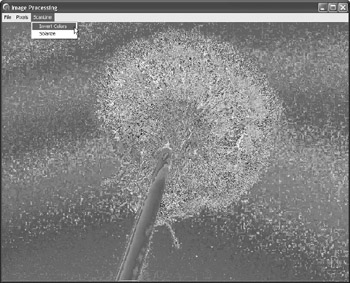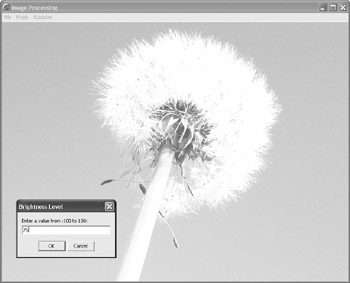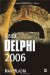The ScanLine Property

The TBitmap.ScanLine property is an indexed property that returns a pointer to a row of pixels. The way we use the result of the ScanLine property differs greatly, depending on the bitmap's format. To determine or specify the format of a TBitmap, use its PixelFormat property. The PixelFormat property is a TPixelFormat enumeration, declared in the Graphics unit:

TPixelFormat = (pfDevice, pf1bit, pf4bit, pf8bit,    pf15bit, pf16bit, pf24bit, pf32bit, pfCustom);

If the bitmap's PixelFormat doesn't match the way we access the bitmap's pixels, the result is usually very ugly. For instance, the following figure shows an Invert Colors effect that failed because the code tried to treat a pf8bit bitmap as if it were a pf24bit bitmap.Figure 28-4: This happens when the bitmap's PixelFormat and code aren't compatible

Since this is not a graphics book, we'll only be using the standard 24-bit (true-color) bitmaps. To achieve this, we have to change the PixelFormat of the image after it's loaded to pf24bit. So, here's how the File ® Open command should look:

procedure TMainForm.FileOpenClick(Sender: TObject); begin   if OpenDialog.Execute then   begin     FImage.LoadFromFile(OpenDialog.FileName);     { always treat the bitmap as a 24-bit bitmap }     FImage.PixelFormat := pf24bit;     Invalidate; { Display the image }   end; end;

As you already know, each pixel in a 24-bit bitmap is described by three values of red, green, and blue. What you might have forgotten is that these values are stored in reverse order in memory. The pixel's blue component is stored first, then the green, and finally the red component. So, when you use the ScanLine property to access a row of pixels in an image, you get a pointer to an array of bytes that looks like this:

BlueGreenRed|BlueGreenRed|BlueGreenRed|BlueGreenRed| BlueGreenRed…

To get the above result, you have to assign the value of the ScanLine property to a PByteArray variable, which enables us to treat a memory block as if it were an array:

var   p: PByteArray; begin     p := FImage.ScanLine; end;

The following example uses the ScanLine property to access the first row of pixels and then sets the first pixel to black and the second pixel to white.

procedure TMainForm.ChangeTwoPixels(Sender: TObject); var   p: PByteArray; begin   p := FImage.ScanLine;   { change first pixel to clBlack }   p := 0;   p := 0;   p := 0;   { change second pixel to clWhite }   p := 255;   p := 255;   p := 255;   Invalidate; end;

When you use the PByteArray type to access the pixels in an image, the first pixel is located at offset 0, the second at offset 3, the third at offset 6, the fourth at offset 9, and so on. So if you want to modify all pixels in a row, you can't use the following code because it only changes the first third of the pixels to black:

procedure TMainForm.ChangeTwoPixels(Sender: TObject); var   p: PByteArray;   x: Integer; begin   p := FImage.ScanLine;   for x := 0 to Pred(FImage.Width) do     p[x] := 0;   Invalidate; end;

To correctly access the x pixel, you have to multiply it by 3 to get its offset in the scan line. The byte at location x*3 is actually the pixel's blue component. The pixel's green component is located at offset x*3+1 and the pixel's red component is located at offset x*3+2.

So, here's what you have to write in order to change all pixels in an image to black:

procedure TMainForm.Blackness(Sender: TObject); var   p: PByteArray;   x: Integer;   y: Integer; begin   { loop through all lines }   for y := 0 to Pred(FImage.Height) do   begin     p := FImage.ScanLine[y];     for x := 0 to Pred(FImage.Width) do     begin       p[x*3] := 0;       p[x*3+1] := 0;       p[x*3+2] := 0;     end;      // for x   end;       // for y   Invalidate; end;

Now that you know how to use the ScanLine property, you can write new versions of the Invert Colors and Solarize effects to see the enormous speed difference gained by using the ScanLine property and the PByteArray type.

Here's the ScanLine version of the Invert Colors effect:

procedure TMainForm.ScanLineInvertColorsClick(Sender: TObject); var   p: PByteArray;   x: Integer;   y: Integer; begin   for y := 0 to Pred(FImage.Height) do   begin     { get the pointer to the y line }     p := FImage.ScanLine[y];     for x := 0 to Pred(FImage.Width) do     begin       { modify the blue component }       p[x*3] := 255 - p[x*3];       { modify the green component }       p[x*3+1] := 255 - p[x*3+1];       { modify the red component }       p[x*3+2] := 255 - p[x*3+2];     end;      // for x   end;       // for y   Invalidate; { display the image } end;

Here's the ScanLine version of the Solarize effect:

procedure TMainForm.ScanLineSolarizeClick(Sender: TObject); var   p: PByteArray;   x: Integer;   y: Integer; begin   for y := 0 to Pred(FImage.Height) do   begin     p := FImage.ScanLine[y];     for x := 0 to Pred(FImage.Width) do     begin       if p[x*3] > 127 then         p[x*3] := 255 - p[x*3];       if p[x*3+1] > 127 then         p[x*3+1] := 255 - p[x*3+1];       if p[x*3+2] > 127 then         p[x*3+2] := 255 - p[x*3+2];     end;      // for x   end;       // for y   Invalidate; end;

Before you start creating a bunch of routines that use the PByteArray type, you might want to consider creating your own pixel description type. You can, for instance, use the following type and array to treat the pixels in the image as an array of TMyPixelDescriptor records:

type   { the order of fields must match the BGR order of bytes in memory }   TMyPixelDescriptor = record     Blue: Byte;     Green: Byte;     Red: Byte;   end;   PMyPixelArray = ^TMyPixelArray; { use an array of 32768 pixels if you want to make sure   you'll be able to use extra large images sometime in the future } TMyPixelArray = array[0..32767] of TMyPixelDescriptor;

Here's the much more readable Solarize effect written using the PMyPixelArray type:

procedure TMainForm.ScanLineSolarizeClick(Sender: TObject); var   p: PMyPixelArray;   x: Integer;   y: Integer; begin   for y := 0 to Pred(FImage.Height) do   begin     p := FImage.ScanLine[y];     for x := 0 to Pred(FImage.Width) do     with p[x] do     begin       if Blue > 127 then Blue := 255 - Blue;       if Green > 127 then Green := 255 - Green;       if Red > 127 then Red := 255 - Red;     end;      // with p[x]   end;       // for y   Invalidate; end;

Converting the Image to Grayscale

One of the easiest ways to convert a color image to grayscale is to use the following formula:

Gray = (Red * 3 + Blue * 4 + Green * 2) div 9

When you get the gray value, you have to assign it to all three components. Here's the entire conversion to grayscale, again using the PMyPixelArray type:

procedure TMainForm.ScanLineGrayscaleClick(Sender: TObject); var   p: PMyPixelArray;   x: Integer;   y: Integer;   gray: Integer; begin   for y := 0 to Pred(FImage.Height) do   begin     p := FImage.ScanLine[y];     for x := 0 to Pred(FImage.Width) do     with p[x] do     begin       gray := (Red * 3 +  Blue * 4 +  Green * 2) div 9;       Blue := gray;       Red := gray;       Green := gray;     end;      // with p[x]   end;        // for y   Invalidate; end;

Adjusting the brightness of an image is likewise a pretty easy task. To darken or lighten the image, you can use the following formula:

NewPixel = OldPixel + (1 * Percent) div 100

To darken the image, pass a negative Percent value; to lighten it, pass a positive Percent value. If you pass –100 as the Percent, you'll get a black image, and if you pass +100 as the Percent value, you'll get a white image. If you want your brightness algorithm to produce the same output as, for instance, Adobe Photoshop or other image editors, simply divide the NewPixel value by 200 to halve the user's ability to darken or lighten the image:

NewPixel = OldPixel + (1 * Percent) div 200

To properly implement the Adjust Brightness effect, you have to create a simple function that will make sure the new values are in the allowable 0 to 255 range:

function IntToByte(AInteger: Integer): Byte; inline; begin   if AInteger > 255 then     Result := 255   else if AInteger < 0 then     Result := 0   else     Result := AInteger; end;

You should also implement the effect as a separate method that accepts the Percent parameter and then call this method inside the ScanLine ® Adjust Brightness command's OnClick event handler:

{ Percent must be a number from -100 to 100 } procedure TMainForm.AdjustBrightness(Percent: Integer); var   p: PMyPixelArray;   x: Integer;   y: Integer;   amount: Integer; begin   amount := (255 * Percent) div 200;   for y := 0 to Pred(FImage.Height) do   begin     p := FImage.ScanLine[y];     for x := 0 to Pred(FImage.Width) do     with p[x] do     begin       Blue := IntToByte(Blue + amount);       Green := IntToByte(Green + amount);       Red := IntToByte(Red + amount);     end;   end;   Invalidate; end;

Finally, to enable the user to enter a value from –100 to 100 before calling AdjustBrightness, the OnClick event handler calls the InputBox function, which displays a small input dialog box and allows the user to enter a string value:

procedure TMainForm.ScanLineBrightnessClick(Sender: TObject); var   amount: Integer; begin   amount := StrToInt(InputBox('Brightness Level',     'Enter a value from -100 to 100:', '50'));   AdjustBrightness(amount); end;

The following figure shows both the input dialog box and the result of passing 75 to the AdjustBrightness method.Figure 28-5: Adjusting the brightness of the imageInside Delphi 2006 (Wordware Delphi Developers Library)
ISBN: 1598220039
EAN: 2147483647
Year: 2004
Pages: 212